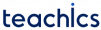# SR latch

A latch is a temporary storage element that has two stable states (bistable). They operate in signal levels rather than signal transitions. An active-HIGH input SR latch is formed with two cross-coupled NOR gates and an active-LOW inputlatch is formed with two cross-coupled NAND gate.

An SR Latch with two cross-coupled NOR gate is shown in the figure. It has two input S for SET and R for RESET and two outputsand.

## Working of an SR Latch

When S=1 the output of G2 will be 0 (the output of a NOR gate is 0 when any of its input are 1). Since this outputis connected back to an input of G1, and the R input is also 0, the output of G1 must be 1. This output 1 is coupled back to an input of G2, making its outputremain 1 even when the 1 on the S input is removed(Memory State). When the Q output is HIGH, the latch is said to be in the SET state.then&When R=1 and S=0, the output of G1 becomes 0. This 0 on the Q output is coupled back to an input of G2, and since the S input is 0, the output of G2 becomes 1. This 1 on theoutput is then coupled back to an input of G1, making the output Q remains 0 even when the 1 on the R input is removed(Memory State). We can now say that the latch is in the RESET state.then&When 1s are applied to both S and R at the same time, an invalid condition occurs in the operation of an SR latch. As long as the 1s are held simultaneously on the inputs, both theandoutputs are forced to become 0. It will violate the basic complementary operation of the outputs. Also, if the 1s are released simultaneously, both outputs will attempt to go 1. In this situation, you cannot reliably predict the next state of the latch.

This operation is summarized in the following truth table.0 0 NC NC Memory State
0 1 0 1 RESET
1 0 1 0 SET
1 1 Not Used.

## SR Latch using NAND gate

An SR latch can be also constructed by cross-coupling two NAND gates as shown in the figure.

The operation is described in the following truth table.